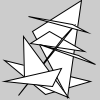# Py5Shape.vertices()#

Create a collection of vertices.

## Examples#import numpy as np

def setup():
random_triangle_vertices = 100 * np.random.rand(25, 2)
s = py5.create_shape()
with s.begin_shape(s.TRIANGLES):
s.vertices(random_triangle_vertices)
py5.shape(s)


## Description#

Create a collection of vertices. The purpose of this method is to provide an alternative to repeatedly calling Py5Shape.vertex() in a loop. For a large number of vertices, the performance of vertices() will be much faster.

The coordinates parameter should be a numpy array with one row for each vertex. There should be two or three columns for 2D or 3D points, respectively.

## Signatures#

vertices(
coordinates: npt.NDArray[np.floating],  # 2D array of vertex coordinates with 2 or 3 columns for 2D or 3D points, respectively
/,
) -> None


Updated on June 26, 2023 01:48:37am UTC